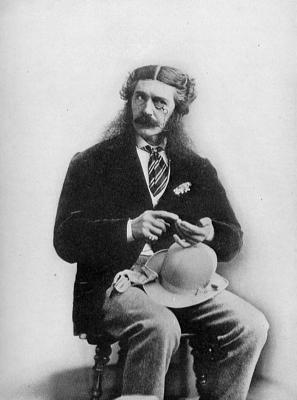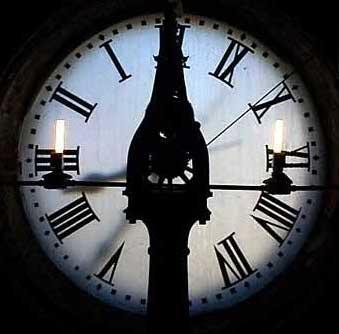# How long does it take to count to one trillion?

Posted by: Gary Ernest Davis on: February 16, 2010

Where does this estimate come from?

Recall that a trillion is a thousand billion, and a billion is a thousand million.

There are Â 31,536,000 seconds in a 365 day year, and one trillion divided by 31,536,000 is approximately 31,710.

So it looks like that’s where the estimate of over 31,000 years to count to one trillion came from: it’s based on an assumption that a person is counting one number each second.Can we easily count to 10 in 10 seconds? Sure: just say each number name, taking at most one second each time: “one”, “two”, …, “ten”. I had no trouble doing this.

How about counting to 100? Could we do that in 100 seconds by saying each number name in 1 second or less?

Now, it’s not quite so clear. The problem is the longer number names as we get into the teens and then the twenties, thirties, forties, and so on. Names like “thirteen, fourteen, fifteen, …” take longer to say than the number names from 1 through 10. And when we get to 20 we have “twenty one, twenty two, twenty three” and so on.

So unless we speed up how fast we say each number name, it’s looking like it will take longer than 100 seconds to count to 100. How much longer?### Counting time assumptions

Let’s try to model how long it will take to count to 100 and then to 1,000, by making some simplifying, but moderately realistic, assumptions.

Let’s assume that we can say each single word in a name in 1 second.

This is not exactly correct, because it takes longer to say “nineteen” than it does to say “ten”. But what we are assuming is that it will take twice as long to say “twenty one”, and three times as long to say “one hundred one”.

Note, by the way, that the answer depends on where one lives. First of all, even in English, people in the UK and in Commonwealth countries do not count like people in the United States, and people in Vietnam and Germany count differently again, and from each other. I am assuming US English counting.

### Counting to 100

The numbers 1 through 20 all Â take 1 second to say, as do the 7 numbers 30, 40, 50 ,60, 70, 80, and 90, while the remaining 73 numbersÂ  up to 100 all take 2 seconds to say.

These are approximations, but they serve us better than assuming all numbers take the same time to say.

So our estimated time to count to 100 is now$20+ 7 + 2\times 73 =173$ seconds.

Of course we can do if faster if we speed it up, but there’s a long way to go to one trillion so let’s keep an even pace. At the end we can think about what would happen if we could say more number words each second.

Notice that 173 seconds is close to the time it would take – 180 seconds – to count 90% of the numbers 1 through 1,00 at 2 seconds each.

### Counting to 1,000

With our stated assumptions on how long it takes to say number names, how long will it take us to count to 1,000?

Many of the numbers from 101 through 1,000 will take 4 seconds to say: numbers like 131 (“one hundred thirty one“) and 273 (“two hundred seventy three“).

But there are exceptions.

I tried to take into account all exceptions in counting to 1,00 and, below, in counting to 10,000. To the best of my knowledge I did not omit any exceptions.

Some numbers from 101 through 1,000 will take 2 seconds to say. These are the 9 numbers 200 (“two hundred“), 300, 400, 500, 600, 700, 800 , 900 and 1,000 (“one thousand“).

Some numbers from 101 through 1,000 will take 3 seconds to say. There are a lot more of these.

Between 101 and 199 these are the 27 numbers 101 (“one hundred one“) through 120 (“one hundred twenty“) and 130, 140, 150, 160, 170, 180 ,190.

Similarly between 210 and 299 there are 27 numbers that take 3 seconds to say.

The same goes for 310 to 399, 410 to 499, 510 to 599, 610 to 699, 710 to 799, 8210 to 899 and 910 to 999.

So there are$9\times 27 = 243$ numbers that will take 3 seconds to say.

This leaves 648 numbers between 101 and 1,000 that will take 4 seconds to say.

So the total time to say all the numbers up to 1,000 we estimate as$173+2\times 9+ 3\times 243+4\times 648 =3512$ seconds, which is just under 1 hour.

Notice that this is not far off the number we would get if just assumed we counted 90% of theÂ  numbers up to 1,000 at 4 seconds each.

### Counting to 10,000

How long will it take us to count to 10,000?

Most numbers will take 6 seconds to say: numbers like 1274 (“one thousand two hundred seventy four“), but again there are exceptions.

Let’s count these exceptions to see how far off would be an estimate of$0.9\times 10,000 \times 6 = 5,400$ seconds.

We already know the exceptions up to 1,000, so let’s look at them from 1,001 on.

There are 9 numbers from 1,001 through 10,000 that require only 2 seconds to say. These are: Â 2,000, 3,000; 4,000; 5,000; 6,000; 7,000; 8,000; 9,000 and 10,000.

There are 252 numbers between 1,001 and 10,000 that require 3 seconds to say. These are 1,001 through 1,020; 1,030; 1,040; 1,050 1,060; 1,070; 1,080; 1,090 and the corresponding numbers in the n,000 range where n=2, 3, …, 9.

There are 648 numbers in the range 1,001 through 10,000 that require 4 seconds to say. These are 1,021 through 1,029; 1,031 through 1,039; … , 1,091 through 1,099 and the corresponding numbers in the$n\times 1,000$ range where$n=2, 3, \ldots, 9$.

There are$9\times 243=2187$ numbersÂ  in the range 1,001 through 10,000 that require 5 seconds to say. These are the numbers$n\times 1,000+m$ where$n=1,2,\ldots 9$ and$1\leq m\leq 999$ is a number that takes 3 seconds to say. Â For each choice of$n=1,2,\ldots 9$ there are 243 of the latter, as we saw in counting up to 1,000.

The remaining 5,913 numbers in the range 1,001 through 10,000 take 6 seconds to say.

So the total time to count to 10,000 is$3512+2\times 9+3\times 252+4\times 648+5\times 2187+ 6\times 5,913 = 53,291$ seconds.

Again, this is close to the time we would take – 54,000 -Â  if we counted 90% of the numbers up to 10,000 at 6 seconds each.

### An estimate of the time to count to one trillion

Counting all exceptions as we say larger numbers becomes more difficult.

However by now we can see a glimmer of a general pattern: there are many more numbers from 100 billion to one trillion Â than there are from one to 100 billion. In fact there are 9 times as many. So by looking only at numbers from 100 billion to one trillion we are covering 90% of all the numbers from one through one trillion.

Most numbers up to one trillion take 19 seconds to say: numbers like 832, 521,432,987 (“eight hundred thirty two billion, five hundred twenty one million, four hundred thirty two thousand, nine hundred eighty seven“).

Our general observation is that to count up to$10^n$ takes about as long as it would to count 90% of the numbers up to$10^n$ at the typical rate.

If this continues to hold good up to one trillion then we estimate to count to one trillion would take about$0.9\times 19=17.1$ times as long as if we were to count one number per second.

That is, we estimate it would take approximately 542,241 years to count to one trillion.

### What if we could count faster?

Let’s imagine we can say 3 number words each second (as in: “one one thousand”). Still it would take us something like 180,747 years to count to one trillion.

### How far could we expect to count in a lifetime?

If we counted from age 5 through 85, 80 years in total , saying one number word each second, with 8 hours a day off Â for sleeping, eating and bathroom breaks, how far could we count in those 4,608,000 seconds? Less than one million?

### 3 Responses to "How long does it take to count to one trillion?"I’m exhausted reading your post! It’s late here – is it ok if I just count a couple of dozen sheep?Reading this post, it occurs to me that the English language has a rather inefficient system for saying large numbers.

In Lojban, the number 1337 would not be said like “one thousand three hundred thirty seven” but more like a chain of digits “onethreethreeseven”.

What if we do that, and let every number contain one syllable?

We could even take it further, and let’s say there are 1200 distinguishable human syllables (not researched, just a random guess), we could use a base 1200 system. In this system, any number in the 100-billion to 1-trillion range would take up 3 syllables.

If one could say these syllables extremely quickly it would cut down the time considerably, perhaps even enough to cut down the time to count to a trillion to one lifetime?[…] (Source) Posted in OMG Facts Tags: general facts, maths facts, OMG Facts, one trillion « Website founder Mark Zuckerberg cannot be blocked on Facebook. You can leave a response, or trackback from your own site. […]